DOC HOME SITE MAP MAN PAGES GNU INFO SEARCH PRINT BOOK
Sets - Sets(C++)

# Internal representation of Sets

Internally, Bags, Sets, and pointer sets are represented as digital tries. An empty pointer set is represented by a single null pointer.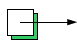A pointer set containing one pointer is represented by the pointer itself. Suppose the pointer is 0x264a8: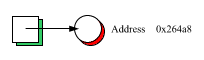The data structure starts to get interesting when we add a second pointer. A node containing sixteen pointers is allocated, and the existing pointer is moved into cell i, where i is the low-order hex digit of the node -- in this case, cell 8:

NOTE: The examples in this section assume 32-bit addresses and (for Sets and Bags) 32-bits in the type Set_or_Bag_hashval. The representation may be different on your machine.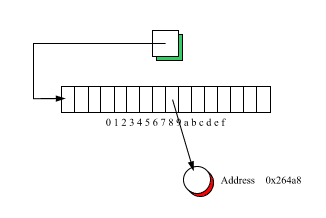The rightmost hex digit of the new pointer similarly determines which cell in the node it will be stored in. Let's say the new pointer's value is 0x254b4; then the pointer will be stored in cell 4: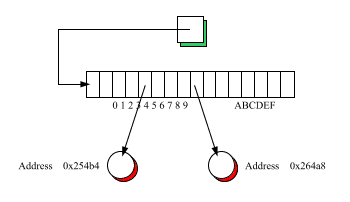Obviously, this is the serendipitous case. What if the low-order hex digits had been the same? To see what happens when pointers collide, consider inserting the pointer 0x26508. Cell 8 is occupied, so the entire process is repeated: a new node is allocated and the existing pointer (0x264a8) is moved down into cell a of the new node, the cell being determined this time by the second hex digit: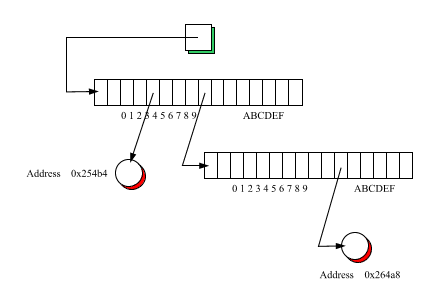Similarly, the new pointer (0x26508) is stored into cell 0 of the new node, based on its second hex digit.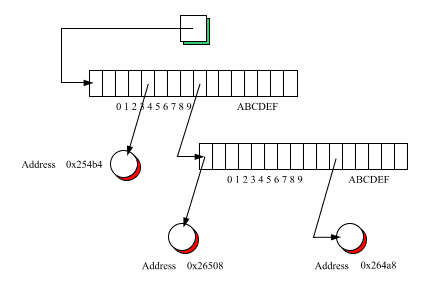As pointers are added, a 16-ary tree develops. The tree has the following invariant: for a leaf at level N, the low-order N hex digits trace out the sequence of cell indices by which the leaf is reached from the root.

Note three important consequences of this scheme (assuming 32-bit pointers):

• The tree can grow to a maximum of eight levels

• It is therefore possible to determine whether the tree contains a given pointer in a maximum of eight steps; testing for containment is therefore O(1).

• The null pointer cannot be stored in the tree because zero means an ``empty'' cell in this scheme.
A Set, unlike a pointer set, is a container for objects, not pointers to objects. Set uses the same basic data structure as pointer set, but with a twist: the hash value is used to determine the insertion point in the tree. To illustrate, suppose we have a Set<String>, and we insert the Strings "Dan", "George", and "Barbara". Suppose further that "Dan" hashes to 0x264a8, "George" hashes to 0x254b4, and "Barbara" hashes to 26508. Then the data structure would look like this: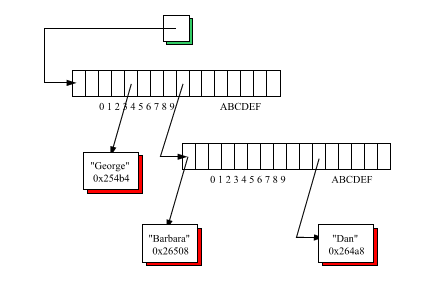Note that the hash value is saved to avoid recomputing it when existing elements must be moved down into a new node.

The actual data structure is actually somewhat more complicated because of the possibility of hash collisions. A collision occurs whenever two elements hash to the same value, and therefore vie for the same location in the data structure. The solution is to have the leaf nodes contain lists of objects, rather than the objects themselves. For example, suppose we insert the String "Mikhail", and it hashes to 02254b4; then "George" and "Mikhail" will occupy a list of length 2: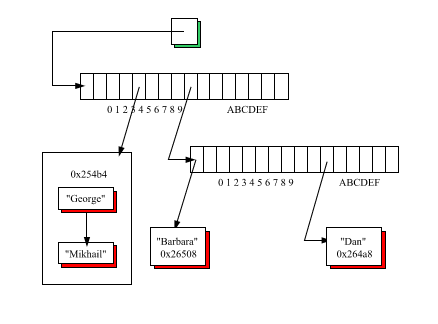The data structure for Bags requires a simple extension to this data structure; the number of occurrences is stored together with each element value. Suppose that we were to store two more occurrences of "George", two more occurrences of "Mikhail", and three more occurrences of "Dan". Then the Bag data structure would look like this: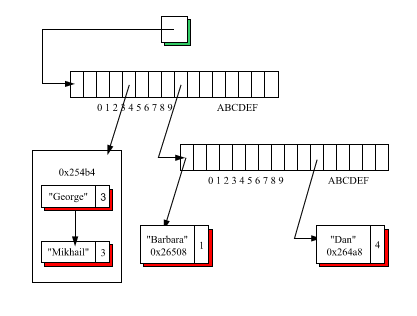For a perfect hash function, no collision list will contain more than one element; for a good hash function (like the one developed for String), most lists will contain one element, a few will contain two elements, still fewer will contain three, and so-on. When you fail to provide any hash function at all, there will be a single collision list containing all the elements of the Set: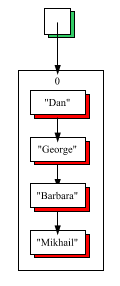Next topic: Symbol(C++) as an application of sets
Previous topic: Modifying elements in place